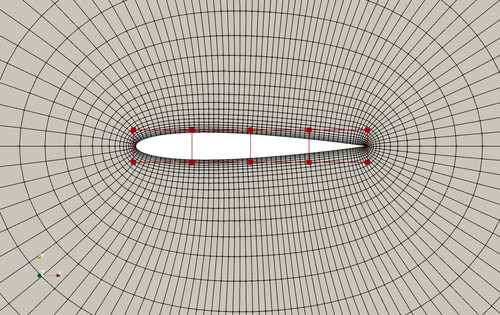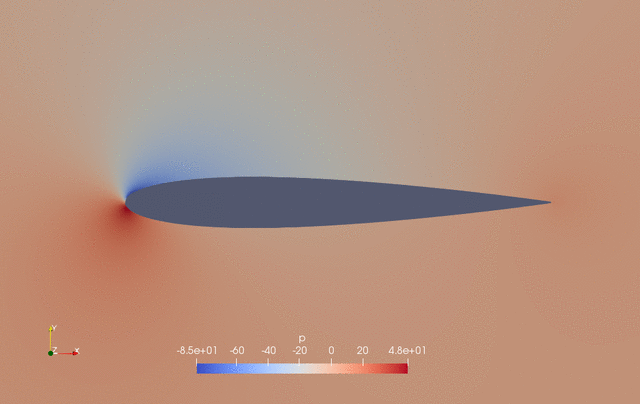The following is a multipoint aerodynamic shape optimization case for the NACA0012 airfoil at low speed. We optimize the weighted drag coefficient considering three different flight conditions, i.e., CL=0.3, 0.7, and 0.5 (nominal). The weight of these three conditions are 0.25, 0.25, and 0.5, respectively.

```Case: Airfoil aerodynamic optimization
Geometry: NACA0012
Objective function: Weighted drag coefficient (CD)
Lift coefficient (CL): 0.3, 0.7, and 0.5 (nominal)
Weights: 0.25, 0.25, 0.5 (nominal)
Design variables: 20 free-form deformation (FFD) points moving in the y direction, three angle of attacks
Constraints: Symmetry, volume, thickness, and lift constraints (total number: 34)
Mach number: 0.02941 (10 m/s)
Reynolds number: 0.6667 million
Mesh cells: ~4,000
Solver: DASimpleFoam
```Fig. 1. Mesh and FFD points for the NACA0012 airfoil

The `runScript.py` is based on the one used in the NACA0012 low speed case with the following modifications:

• In the global parameters, we define the number of multipoint configurations `nMultiPoints = 3`, and set far field boundary conditions and flight conditios for all the three configurations. `MPWeights`, `U0`, `alpha0`, and `CL_target` are the weights, far field velocity, angle of attack, and target lift coefficient for the three configurations. Note that we set the nominal conditions `CL_target = 0.5` as the last element in the list such that the intermdiate flow fields will be saved for this condition, which facilitates the post-processing using Paraview.

``````# global parameters
nMultiPoints = 3
MPWeights = [0.25, 0.25, 0.5]
U0 = [10.0, 10.0, 10.0]
# we use the first U0 as reference velocity to normalize CD and CL
URef = U0
CL_target = [0.3, 0.7, 0.5]
alpha0 = [3.008097, 7.622412, 5.139186]
p0 = 0.0
nuTilda0 = 4.5e-5
A0 = 0.1
``````
• In `daOptions` we set `"multiPoint": True` to activatve the multipoint optimization mode, and set `"nMultiPoints": nMultiPoints`.

• In the design variable setup section, we add three angle of attack design variables, each for one flight condition. We also append `mp0_`, `mp1_`, and `mp2_` to the name of the angle of attack variable. Note: we provide a dummy function (`dummyFunc`) for alpha variable. This is because we will use a function `setMultiPointCondition` to change the angle of attack later in `runScript.py`, so there is no need to provide an alpha function for `DVGeo.addGeoDVGlobal` here.

``````for i in range(nMultiPoints):
DVGeo.addGeoDVGlobal("mp%d_alpha" % i, alpha0[i], dummyFunc, lower=0.0, upper=10.0, scale=1.0)
daOptions["designVar"]["mp%d_alpha" % i] = {
"designVarType": "AOA",
"patch": "inout",
"flowAxis": "x",
"normalAxis": "y",
}
``````
• In the optFuncs setup section. We provide a `setMultiPointCondition` function to set flow boundary conditions for the three flight configurations. Here `setMultiPointCondition` uses the design variable dictionary `xDV` and the multipoint `index` as the input, and it implements a general method to change the boundary conditions. For this case, we first extract the alpha value from `xDV`, compute the far field velocity components, replace the `primalBC` key in DAOption and update its value in DASolver (`DASolver.updateDAOption()`).

``````def setMultiPointCondition(xDV, index):
aoa = xDV["mp%d_alpha" % index].real * np.pi / 180.0
inletU = [float(U0[index] * np.cos(aoa)), float(U0[index] * np.sin(aoa)), 0]
DASolver.setOption("primalBC", {"U0": {"variable": "U", "patch": "inout", "value": inletU}})
DASolver.updateDAOption()
return
``````
• Next, we define a function (`setMultiPointObjFuncs`) to combine the objective function values. This function will be used in optFuncs.calcObjFuncValuesMP.

``````def setMultiPointObjFuncs(funcs, funcsMP, index):
for key in funcs:
if "fail" in key:
pass
elif "DVCon" in key:
funcsMP[key] = funcs[key]
elif "CD" in key:
try:
funcsMP["obj"] += funcs[key] * MPWeights[index]
except Exception:
funcsMP["obj"] = 0.0
funcsMP["obj"] += funcs[key] * MPWeights[index]
elif "CL" in key:
funcsMP["mp%d_CL" % index] = funcs[key]
return
``````

In optFuncs.calcObjFuncValuesMP, we loop over all the flight conditions and call `DASolver()` to solve the primal and compute the objective dict `funcs`. Then we call `setMultiPointObjFuncs`, which takes `funcs` and multipoint `index` as input, and outputs the dict `funcsMP`, where `MP` means multipoint. `funcs` contains the objective and constraint function values and a `fail` flag. Here we create a `obj` key by combining `CD` computed from each flight condition. An example is as follows.

``````funcs   = {"CD": 0.01, "CL": 0.3, "DVCon_0": 1.0, "fail": False} # flight condition 0
funcs   = {"CD": 0.03, "CL": 0.7, "DVCon_0": 1.0, "fail": False} # flight condition 1
funcs   = {"CD": 0.02, "CL": 0.5, "DVCon_0": 1.0, "fail": False} # flight condition 2
# weights for the three flight conditions are 0.25, 0.25 and 0.5
funcsMP = {"obj": 0.02, "mp0_CL": 0.3, "mp1_CL": 0.7, "mp2_CL": 0.5, "DVCon_0": 1.0, "fail": False} # funcs for multipoint
``````

Note that we do not combine CL, instead we keep all the lift coefficients by appending `mp0_`, `mp1_`, and `mp2_` to the objective function names. This will allow us to set lift constraints for all flight conditions (see `optProb.addCon` in `runScript.py`). Also note that the geometry constraints (`DVCon`) are same for all three flight conditions, therefore there is no need to do weighting or appending any prefix names to `DVCon`.

• In addition, we define a function `setMultiPointObjFuncsSens` to combine the objective function derivatives. This function will be used in optFuncs.calcObjFuncSensMP. In optFuncs.calcObjFuncSensMP, we loop over all the flight conditions and call `DASolver.solveAdjoint()` to solve the adjoint and compute the objective derivative dict `funcsSens`. Then `setMultiPointObjFuncsSens` takes design variable dict `xDVs`, the mulitpoint objective value dict `funcsMP`, objective derivative dict `funcsSens` for flight condition `index` as input, and outputs the multipoint objective derivative dict `funcsSensMP`.

``````def setMultiPointObjFuncsSens(xDVs, funcsMP, funcsSens, funcsSensMP, index):
for key in funcsMP:
try:
keySize = len(funcsMP[key])
except Exception:
keySize = 1
try:
funcsSensMP[key]
except Exception:
funcsSensMP[key] = {}

if "fail" in key:
pass
elif "DVCon" in key:
funcsSensMP[key]["mp%d_alpha" % index] = np.zeros((keySize, 1), "d")
funcsSensMP[key]["shapey"] = funcsSens[key]["shapey"]
elif "obj" in key:
funcsSensMP[key]["mp%d_alpha" % index] = funcsSens["CD"]["mp%d_alpha" % index] * MPWeights[index]
try:
funcsSensMP[key]["shapey"] += funcsSens["CD"]["shapey"] * MPWeights[index]
except Exception:
funcsSensMP[key]["shapey"] = np.zeros(len(xDVs["shapey"]), "d")
funcsSensMP[key]["shapey"] += funcsSens["CD"]["shapey"] * MPWeights[index]
elif "mp%d_CL" % index in key:
for alphaI in range(nMultiPoints):
if alphaI == index:
funcsSensMP[key]["mp%d_alpha" % alphaI] = funcsSens["CL"]["mp%d_alpha" % index]
else:
funcsSensMP[key]["mp%d_alpha" % alphaI] = np.zeros((keySize, 1), "d")
funcsSensMP[key]["shapey"] = funcsSens["CL"]["shapey"]

return
``````

The objective derivative dict `funcsSens` contains the derivatives of all objectives and constraints with respect to all design variables.

For `DVCon`, we need to copy the value from funcsSens, while setting all alpha derivatives to zeros. This is because the geometry constraint is independent of the angle of attack.

For `obj`, we need to combine the `shapey` derivatives by the weights, and set the derivative for `mp*_alpha` individually.

For each `mp*_CL`, we need to assign `shapey` derivative from each flight condition, and assign `mp*_alpha` that corresponds to this flight condition and zero out the alpha derivatives from other flight conditions. This is because the angle of attack from other flight conditions does not impact `CL` for the current flight condition.

As an example, the `funcsSens` for each condition and the combined `funcsSensMP` may look like this:

``````funcsSens = {
"CD": {"shapey": {0.01, 0.01}, "mp0_alpha": 0.001, "mp1_alpha": 0, "mp2_alpha": 0},
"CL": {"shapey": {0.1, 0.1}, "mp0_alpha": 0.01, "mp1_alpha": 0, "mp2_alpha": 0},
"DVCon_0": {"shapey": {0.1, 0.1}, "mp0_alpha": 0, "mp1_alpha": 0, "mp2_alpha": 0}
} # flight condition 0
funcsSens = {
"CD": {"shapey": {0.03, 0.03}, "mp0_alpha": 0, "mp1_alpha": 0.003, "mp2_alpha": 0},
"CL": {"shapey": {0.3, 0.3}, "mp0_alpha": 0, "mp1_alpha": 0.03, "mp2_alpha": 0},
"DVCon_0": {"shapey": {0.1, 0.1}, "mp0_alpha": 0, "mp1_alpha": 0, "mp2_alpha": 0}
} # flight condition 1
funcsSens = {
"CD": {"shapey": {0.02, 0.02}, "mp0_alpha": 0, "mp1_alpha": 0, "mp2_alpha": 0.002},
"CL": {"shapey": {0.2, 0.2}, "mp0_alpha": 0, "mp1_alpha": 0, "mp2_alpha": 0.02},
"DVCon_0": {"shapey": {0.1, 0.1}, "mp0_alpha": 0, "mp1_alpha": 0, "mp2_alpha": 0}
} # flight condition 2
# weights for the three flight conditions are 0.25, 0.25 and 0.5
funcsSensMP = {
"obj": {"shapey": {0.02, 0.02}, "mp0_alpha": 0.00025, "mp1_alpha": 0.0075, "mp2_alpha": 0.001},
"mp0_CL": {"shapey": {0.1, 0.1}, "mp0_alpha": 0.01, "mp1_alpha": 0, "mp2_alpha": 0},
"mp1_CL": {"shapey": {0.3, 0.3}, "mp0_alpha": 0, "mp1_alpha": 0.03, "mp2_alpha": 0},
"mp2_CL": {"shapey": {0.2, 0.2}, "mp0_alpha": 0, "mp1_alpha": 0, "mp2_alpha": 0.02},
"DVCon_0": {"shapey": {0.1, 0.1}, "mp0_alpha": 0, "mp1_alpha": 0, "mp2_alpha": 0}
} # multipoint function derivatives
``````
• After the above two functions are set, we need to initialize them in `optFuncs`

``````optFuncs.setMultiPointCondition = setMultiPointCondition
optFuncs.setMultiPointObjFuncs = setMultiPointObjFuncs
optFuncs.setMultiPointObjFuncsSens = setMultiPointObjFuncsSens
``````
• Finally, in the optimization task, we need to provide multipoint value and derivative computation functions by setting `objFun=optFuncs.calcObjFuncValuesMP` and `sens=optFuncs.calcObjFuncSensMP`. Also, we need to set three lift constraints

``````for i in range(nMultiPoints):
optProb.addCon("mp%d_CL" % i, lower=CL_target[i], upper=CL_target[i], scale=1)
``````

To run this case, first download tutorials and untar it. Then go to tutorials-main/NACA0012_Airfoil/multipoint and run the `preProcessing.sh` script to generate the mesh:

```./preProcessing.sh
```

Then, use the following command to run the optimization with 4 CPU cores:

```mpirun -np 4 python runScript.py 2>&1 | tee logOpt.txt
```

The case ran for 50 steps and took about 50 minutes using Intel 3.0 GHz CPU with 4 cores. According to `logOpt.txt` and `opt_SLSQP.txt`, the initial combined drag is 0.021973710 and the optimized drag is 0.017867543 with a drag reduction of 18.7%.

The evolution of pressure and shape during the optimization is as follows.Fig. 2. Pressure and shape evolution during the optimization process